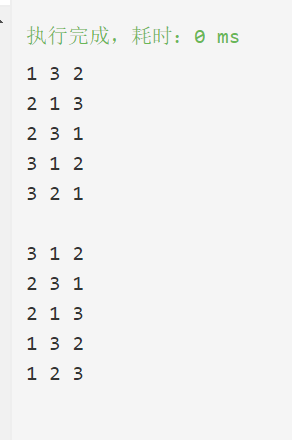## STL中next_permutation()和prev_permutation()函数实现_prev permutation 实现-程序员宅基地

next_permutation()和permutation()是STL提供的用来计算下一个排列和上一个排列的算法；刷题中经常用到，比较有用。

`next_permutation（）`实现

``````template <class iterator>
bool next_permutation(iterator first,iterator last)
{

if(first==last)return false;
iterator i=first;
if(++i==last)return false;
i=last;
--i;
for(;;)
{

iterator ii=i;
--i;
if(*i<*ii)
{

iterator j=last;
while(!(*i<*--j));
swap(*i,*j);
reverse(ii,last);
return true;
}
if(i==first)
{

reverse(first,last);
return false;
}
}
}

``````

`prev_permutation()`实现

``````template <class iterator>
bool prev_permutation(iterator first,iterator last)
{

if(first==last)return false;
iterator i=first;
if(++i==last)return false;
i=last;
--i;
for(;;)
{

iterator ii=i;
--i;
if(*i>*ii)
{

iterator j=last;
while(!(*i>*--j));
swap(*i,*j);
reverse(ii,last);
return true;
}
if(i==first)
{

reverse(first,last);
return false;
}
}
}
``````

``````int main() {

vector<int>temp{
1,2,3};
while( ::next_permutation(temp.begin(),temp.end()))
{

for(auto &c:temp)
cout<<c<<" ";
cout<<endl;
}
temp={
3,2,1};
cout<<endl;
while( ::prev_permutation(temp.begin(),temp.end()))
{

for(auto &c:temp)
cout<<c<<" ";
cout<<endl;
}

return 0;
}
``````### C#如何实现控件移动拖动-程序员宅基地

1 //在picturebox鼠标移动2 private void picBox_MouseMove(object sender, MouseEventArgs e)3 {4 if (MoveFlag)5 {6 picBox.Left += Convert.ToInt16(e.X - xPos);//设置x坐标.7 pic..._c# picbox 修改矩形

### python之错误：OSError: [WinError 10048]-程序员宅基地

OSError: [WinError 10048] 通常每个套接字地址(协议/网络地址/端口)只允许使用一次工具：Pycharm问题：博主在进行一次使用socket模块进行服务端和客户端之间的连接时出现如上错误。错误如下：1：server.py 代码# coding=utf-8"""@author: jiajiknag程序功能：服务器"""import socket# 创建套接字一个实例

### 深度学习pytorch- tensor, dtype 和 shape_获取torch.tensor shape和dtype-程序员宅基地

tensorsnum.代表float类型， 一般只用写一个数字， 代表整体。shape: 矩阵大小float32 数字类型tensor 可以表示数字， 1， 2， 3，…， n 维矩阵。import torch# Numbert1 = torch.tensor(1.)t1tensor(1.)t1.dtypetorch.float32t1.shapetorch.Size([])# Vectort2 = torch.tensor([1., 2, 3, 4])_获取torch.tensor shape和dtype